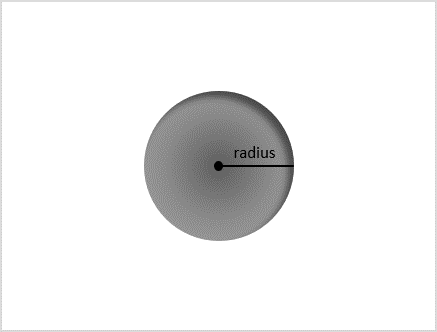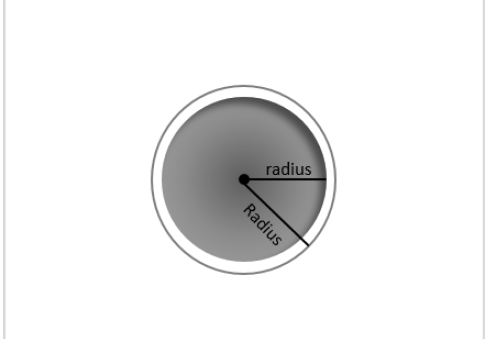# Python Program to calculate the volume and area of Sphere

A sphere (solid) is usually considered a two-dimensional figure even though the figure is seen in three planes from its center. The main reason for this is that, a sphere is only measured using its radius.

However, a hollow sphere is considered a three-dimensional figure since it contains space within its spherical walls and has two different radii to measure its dimensions.The spherical figure only has total surface area since there is only one dimension to measure the entire object. The formula to calculate the surface area of a sphere is −

Area of solid sphere − $\mathrm{{4\pi r^2}}$

The volume of a sphere is considered as the mass held by the circular walls of the object. The formula to calculate volume of a sphere is given as −

Volume of solid sphere − $\mathrm{{\frac{4}{3}\pi r^3}}$

Volume of hollow sphere − $\mathrm{{\frac{4}{3}\pi(R\:-\:r)^3}}$

Where, R is the radius of outer sphere and r is the radius of inner sphere.### Input Output Scenarios

Let us look at some input output scenarios to calculate the area and volume of a sphere −

Assume the area and volume to be found is of a solid sphere −

Input: 7 // 7 is the radius
Result: Area - 314.1592653589793
Volume - 359.188760060433


Assume the area and volume to be found is of a hollow sphere −

Input: (7, 5) // 7 is the outer radius, 5 is the inner radius
Result: Area - 314.1592653589793 // Area is same
Volume - 100.53096491487338


## Using Mathematical Formula

In the python program, we use the discussed mathematical formulae and calculate the area and volume of the sphere. We import the match library to use the pi constant.

### Example

Following is an example to find the area and volume of the spherical 3D figure −

import math

R = 7 #outer radius of sphere
r = 5 # inner radius of sphere

#calculating area of solid sphere
area = 4*(math.pi)*(r)*(r)

#calculating volume of hollow sphere
volume_hollow = 4*(math.pi)*(R - r)*(R - r)*(R - r)

#calculating volume of solid sphere
volume_solid = (1/3)*(math.pi)*(R)*(R)*(R)

#displaying output
print("Area of the sphere: ", str(area))
print("Volume of the hollow sphere: ", str(volume_hollow))
print("Volume of the solid sphere: ", str(volume_solid))


### Output

The output is displayed as given below −

Area of the sphere: 314.1592653589793
Volume of the hollow sphere: 100.53096491487338
Volume of the solid sphere: 359.188760060433


## Function to calculate Area and Volume

Python also makes use of functions to improve modularity of the program. In this case, we use a function that calculates sphere’s area and volume.

### Example

In the following python program, we are calculating the area and volume of both solid and hollow spheres using a user-defined function −

import math
def sphere_area_volume(R, r):
#calculating area of solid sphere
area = 4*(math.pi)*(r)*(r)

#calculating volume of hollow sphere
volume_hollow = 4*(math.pi)*(R - r)*(R - r)*(R - r)

#calculating volume of solid sphere
volume_solid = (1/3)*(math.pi)*(R)*(R)*(R)

#displaying output
print("Area of the sphere: ", str(area))
print("Volume of the hollow sphere: ", str(volume_hollow))
print("Volume of the solid sphere: ", str(volume_solid))

R = 7 #outer radius of sphere
r = 5 # inner radius of sphere
sphere_area_volume(R, r)


### Output

On executing the above code, output is obtained as −

Area of the sphere: 314.1592653589793
Volume of the hollow sphere: 100.53096491487338
Volume of the solid sphere: 359.188760060433